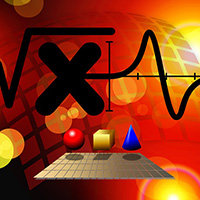Global

Mathematics Experts

Mathematics
IIT Roorkee
India

### Biography

he is working as a Professor in the IIT Roorke in the Department of Mathematics.

#### Research Interest

Real and Complex Analysis,Numerical Analysis, Mathematical Methods ., Approximation Theory, Complex Analysis.q- calculus, (p,q) calculus